# Disarium Number in C#

## Disarium Number in C# with Examples

In this article, I am going to discuss How to Implement Disarium Number Program in C# with Examples. Please read our previous article where we discussed How to Implement the Duck Number Program in C#. Here, in this article, first, we will learn what is a Disarium Number and then we will see how to implement the Disarium Number program using the C# language. And finally, we will see how to print all the disarium numbers between a range of numbers like between 1 to 100 or 100 to 1000, etc.

##### What is a Disarium Number?

A number will be called Disarium if the sum of its digits powered with their respective position is equal to the number itself. For Example: 175 => 1 + 7 + 5 = 1 + 49 + 125 = 175

It will power the number from left to right. The first left element will be of power 1st and 2nd will have power 2 and so on. Other examples of Disarium numbers are 89, 135, 518, etc.

Before going to the solution part, if you have some basic knowledge of what a remainder operator is and how we can use Math.Pow function to get the power of a number, then it will be good for you. As for this question we are going to use these two.

##### Remainder Operator %

The remainder operator % computes the remainder after dividing its left-hand operand by its right-hand operand.

##### Math.Pow(Double, Double) Method

Returns a specified number raised to the specified power.

public static double Pow (double x, double y);

###### Parameters
1. double x: A double-precision floating-point number to be raised to a power.
2. double y: A double-precision floating-point number that specifies a power.
###### Returns
1. double: The number x raised to the power y.’

##### How to Implement Disarium Number in C#?

As we are aware of the remainder operator, we know that its returns the remainder of the two operands. If we apply remainder operator with 10 to any number what we will get? The last digit of the number.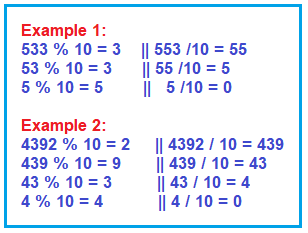We are going to apply the same logic to check whether this number is disarium or not.

Step11: We will extract each digit of the number one by one and apply Math.Pow function to get the power of that respective position and store it into the sum value. As we can clearly see that it will extract from the right side one by one and the rightmost digit will have maximum power. For example 34239 in this particular number, the length of digits is 5, and power 5 will be applied to the rightmost digit that is 9 in this case.

1. Digit 3 will have power 4 because it is in 4th position.
2. Digit 2 will have power 3 because it is in the 3rd position.
3. Digit 4 will have power 2 because it is in the 2nd position.
4. Digit 3 will have power 1 because it is in the 1st position.

So, as we can clearly see the length of the number will be the power for the rightmost digit and as the position of the digit decreasing the power value is also decreasing.

Step2: And at last, we are going to check whether this sum value is equal to the actual given value or not.

Step3: If it is equal to the given number then it is a disarium number otherwise it is not.

##### Disarium Number Program using C#
```using System;
public class Program
{
public static void Main ()
{
Console.WriteLine ("Input a number");
int num = Convert.ToInt32 (Console.ReadLine ());
string numStr = num.ToString ();
int length = numStr.Length;

int divide = 0, sum = 0, copy = num;
while (copy > 0)
{
divide = copy % 10;
sum = sum + (int) Math.Pow (divide, length);
length--;
copy = copy / 10;
}
if (sum == num)
Console.WriteLine ("Disarium Number.");
else
Console.WriteLine ("Not a Disarium Number.");
}
}
```
###### Output: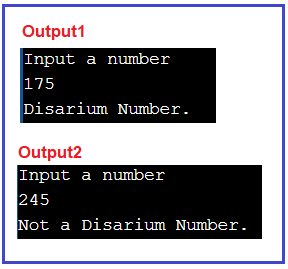TIME COMPLEXITY: 0(n) – where n is the number of digits.

##### Program to print all Disarium numbers between 1 and 100

Here, I am going to print all the Disarium Numbers between 1 and 100. But you can take any number. Let us first see the logic and then we will see the program.

##### CalculateLength Function:

Please have a look at the below image which is going to be our CalculateLength method. The CalculateLength method is used to counts the number of digits present in a number. Here, we are using a while loop to check whether the number is equal to 0 or not. Then Divide the number by 10 and increment the variable-length by 1 and finally Return the length.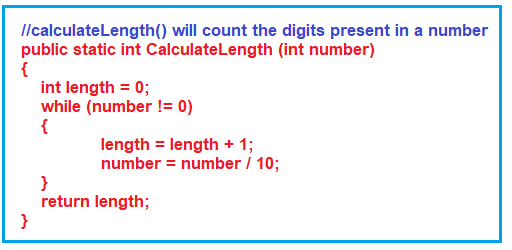##### SumOfDigits Method:

The SumOfDigits method calculates the sum of digits raised based on their respective positions. This method internally makes a call to the CalculateLength method to get the number of digits present in a number and store the value in variable len. Then using a while loop, it calculates the remainder rem repeatedly by dividing the number by 10. Then Calculate the value of rem raised to power its position and store the computed value in the variable sum.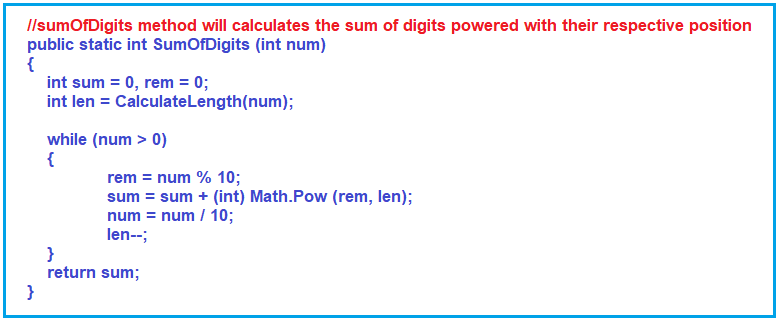##### Main Method:

In order to display all the Disarium numbers between 1 and 100, We start the loop from 1 to 100, then make a call to the SumOfDigits method for each value i.e. from 1 to 100 and store the return value into a variable result. If the value of the result is equal to the number, then the given number is the Disarium number. Here, instead of 1 to 100, you can use any numbers like 100 to 1000, etc.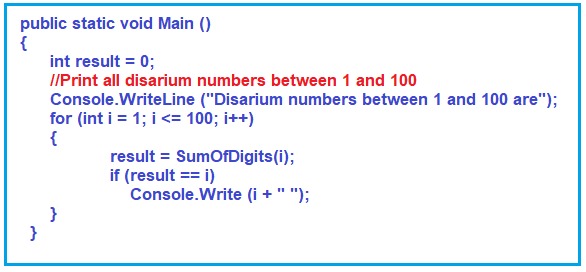###### C# Program to find the Disarium Numbers between 1 and 100.
```using System;
public class DisariumNumbers
{
//CalculateLength method will count the digits present in a number
public static int CalculateLength (int number)
{
int length = 0;
while (number != 0)
{
length = length + 1;
number = number / 10;
}
return length;
}

//SumOfDigits method will Calculates the sum of digits powered with their respective position
public static int SumOfDigits (int num)
{
int sum = 0, rem = 0;
int len = CalculateLength(num);

while (num > 0)
{
rem = num % 10;
sum = sum + (int) Math.Pow (rem, len);
num = num / 10;
len--;
}
return sum;
}

public static void Main ()
{
int result = 0;
//Print all disarium numbers between 1 and 100
Console.WriteLine ("Disarium numbers between 1 and 100 are");
for (int i = 1; i <= 100; i++)
{
result = SumOfDigits(i);
if (result == i)
Console.Write (i + " ");
}
}
}
```
###### Output: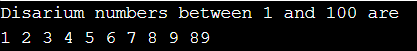In the next article, I am going to discuss the Twisted Prime Number Program in C# with Examples. Here, in this article, I try to explain How to Implement Disarium Number Program in C# with Examples and I hope you enjoy this Disarium Number in the C# with Examples article.